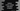# Write a simple calculator program in Python 3## Simple Calculator Program in Python 3 :

In this tutorial, we will learn how to create a Calculator using python 3. The program will read the inputs from the user continuously and based on the user input,it will perform some calculations.

We are going to create only a simple calculator that can perform addition, subtraction, multiplication and division. You can add any other mathematical operations to it if you want.

You can even add GUI to this program using python TKinter. I am not going to cover it in this tutorial, but the process will be the same.

Before going through the code, let me show you how it is going to work :

### The algorithm we are using in this program :

1. We are using one infinite loop to get input from the user continuously. That means a user can use the calculator as many times as he wants. The loop will run an infinite amount of time until the user stops it. We are using one while loop to create this infinite loop.
2. The calculator will exit if the user will enter ‘q’ as an input or the infinite while loop will exit if the user types ‘q’ at the beginning of the loop.
3. For each calculation (add, subtract, multiply, divide) we have different functions. We will call these functions from the main program.
4. Using an ‘if-else’ condition, we are checking what type of calculation the user wants to do.

### Python Program :

``````print("Welcome !!")

# function to add two numbers
print("{} + {} = {}".format(first_num, second_num, first_num + second_num))

# function to substract one number from another
def sub(first_num, second_num):
print("{} - {} = {}".format(first_num, second_num, first_num - second_num))

# function to multiply two numbers
def mul(first_num, second_num):
print("{} * {} = {}".format(first_num, second_num, first_num * second_num))

# function for division
def div(first_num, second_num):
print("{}/{} = {}".format(first_num, second_num, first_num/second_num))

print('''
Enter + for addition
Enter - for Substraction
Enter * for multiplication
Enter / for Division
Enter q to Quit the program
''')

while True:
print("")
user_input = input("Enter calculator type : ")
if user_input == '+':
print("To calculate (number 1 + number 2) :")
add(int(input("Enter number 1 : ")), int(input("Enter number 2 : ")))
elif user_input == '-':
print("To calculate (number 1 - number 2) :")
sub(int(input("Enter number 1 : ")), int(input("Enter number 2 : ")))
elif user_input == '*':
print("To calculate (number 1 * number 2) :")
mul(int(input("Enter number 1 : ")), int(input("Enter number 2 : ")))
elif user_input == '/':
print("To calculate (number 1 / number 2) :")
div(int(input("Enter number 1 : ")), int(input("Enter number 2 : ")))
elif user_input == 'q':
break
print("")
else:
print("Please Enter a Valid Input !!")``````

You can also download this program from here

### Explanation :

1. As you can see in the above program, we have four different functions defined at the beginning of the program. The add function is for adding two numbers, the sub function is for subtraction, the mul function is for the multiplication of two numbers and the div function is for finding out the division.

All of these functions take two numbers as parameters. In this example, we are printing out the result inside each function. You can also return the result from the functions and print them out inside the caller class.

1. Before starting the program, we are printing out one message to the user that + is used for addition, - is for subtraction, * is for multiplication, / is for division and q is to quit the game.

This is a multi-line message and we are using a triple comma for that.

1. The infinite loop is a while loop. ‘while True’ will start that loop for an infinite number of times.
2. Inside the loop, we are using multiple if-elif-else cases. The program will first ask the user to enter the calculation type. It will read it and save it in the user_input variable. For reading this value, we are using the ‘input()’ method.
3. Using the if-elif-else conditions, we are checking the type of the user input. Based on the input type, we are calling the required function defined above.
4. If the input is not valid, the program is printing one message asking the user to enter a valid input. If the input is ‘q’, it will exit.

### Add more Actions to this calculator :

You can add more actions to this calculator program. Just add more functions for each type of calculation and add more if-elif conditions. Happy Coding :)